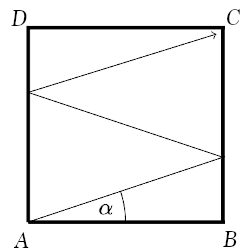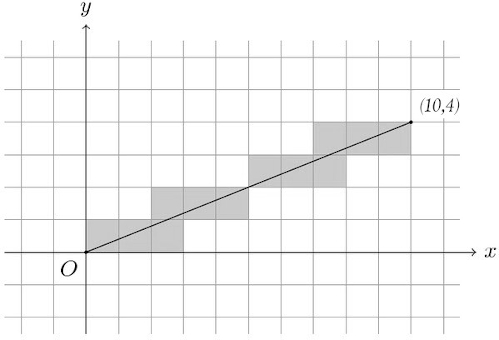# MT #1: Number theory & Trigonometry

## Part A: Short-answer type questions

Submission file: Write answers to all the ten questions on a single sheet of paper. Email a picture of your answer sheet. Name the file as PartA.jpg or PartA.png.

For this part, answers must be written without any explanation.

1. If three numbers $$p$$, $$p+2$$ and $$p+4$$ are primes. What is the value of $$p$$?

2. List all the numbers between 1 and 1000 that are both divisible by 7 and have exactly six factors.

3. Let $$x$$ be a real number such that $$\sec x-\tan x=1/3$$. Evaluate $$\sec x+\tan x$$.

4. Consider the two numbers $$a$$ and $$b$$ whose prime factorizations are as follows: $a = 2\cdot 3\cdot 5^2 \cdot 7^3 \cdot 17^5 \cdot 23$ $b = 2\cdot 3^2\cdot 5 \cdot 7^3 \cdot 11 \cdot 17$
1. Write the prime factorization of the GCD of $$a$$ and $$b$$. [2 marks]
2. Write the prime factorization of the LCM of $$a$$ and $$b$$. [2 marks]

5. Imagine a rectangular carrom board $$ABCD$$ with $$AB=17$$ cm and $$BC=16$$ cm. Assume the striker to be a point moving with no friction. The angle of reflection is equal to the angle of incidence. The striker is shot from point $$A$$ at an angle $$\alpha$$. It bounces off the edges twice and ends up at corner $$C$$ as shown below. What is the value of $$\tan \alpha$$?6. How many ordered pair of integers $$(x,y)$$ satisfy the equation $$\frac{x}{20} = \frac{20}{y}$$?

7. Count the number of ordered pairs $$(a,b)$$ that satisfy two conditions:
• $$a$$ and $$b$$ are relatively prime positive integers
• The product of $$a$$ and $$b$$ is $$10!$$

8. Find the remainder when $$2021^{2021}$$ is divided by 7.

9. Identify the irrational numbers among the three numbers given below.

1. $$\frac{2 \log (3)}{\log (2)+2 \log (3)}$$
2. $$\frac{\log 14}{\log 18}$$
3. $$\sqrt{3} + \sqrt{7} + \sqrt{17} - \sqrt{11}$$

10. Consider the functions $$f_i$$ given below:

\begin{align} f_{1}=(\tan \theta)^{\tan \theta} & \;\; f_{2}=(\tan \theta)^{\cot \theta} \\ f_{3}=(\cot \theta)^{\tan \theta} & \;\; f_{4}=(\cot \theta)^{\cot \theta} \end{align}

Identify the function whose value is greater than others in the domain $$\theta \in \left(0,\pi/4\right)$$.

## Part B: Subjective questions

Submission files: Each question in this part must be answered on a page of its own. Name the files as B1.jpg, B2.jpg, etc. In case you have multiple files for the same question, say B4, name the corresponding files as B4-1.jpg, B4-2.jpg, etc.

Clearly explain your entire reasoning. No credit will be given without reasoning. Partial solutions may get partial credit.

B1. Suppose $$x$$ is an integer. What are the possible values of $$x^{65} \pmod{131}$$ and why?

B2. Consider a coordinate grid made up of horizontal and vertical lines of the form $$x=k$$ and $$y=k$$, where $$k\in \mathbb{Z}$$. Consider a line segment OA where O is the origin and A is the point $$(10,4)$$. Notice that this line segment passes through the interior of 12 cells. In the figure below, the shaded cells are the ones through which the line passes.Suppose a line segment is drawn from the origin O to the point B at $$(48,54)$$. How many cells would would OB pass through?

B3. Suppose $$f(x)=\cos^{-1}(\cos (\pi x))$$. Find the value of $$f(2.6)$$.

B4. Find five unique positive integers $$b_{1}, b_{2}, \cdots, b_{5} \geq 2$$ such that: $\Large \frac{125}{76}=b_{1}-\frac{1}{b_{2}-\frac{1}{b_{3}- \frac{1}{b_4 - \frac{1}{b_5} } }}$

You may find the following fact useful:

Let $$q$$ and $$n$$ be relatively prime positive integers such that $$1 < q < n$$. There exist unique integers $$k, r$$ such that $$n=kq-r, 0 \leq r< q$$.

B5. Find all the positive integer solutions to the following equation: $xyz-xy-yz-xz+x+y+z=52$ Express the solutions as triplets $$(x,y,z)$$.

B6. Prove that there are no integers $$x>0$$ and $$n>1$$ such that $$x(x+1) = 2442^n$$.